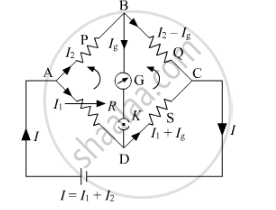# Use Kirchhoff'S Rules to Obtain Conditions for the Balance Condition in a Wheatstone Bridge. - Physics

Use Kirchhoff's rules to obtain conditions for the balance condition in a Wheatstone bridge.

#### Solution

Let us consider a Wheatstone bridge arrangement as shown below.Applying Kirchhoff's loop law to the closed loop ADBA, we get

I1RIgGI2P=0 .....(1)

Here, G is the resistance of the galvanometer.

Applying Kirchhoff's loop law in the closed loop BDCB, we get

IgG+(I1+Ig)S(I2Ig)Q=0.....(2)

When the Wheatstone bridge is balanced, no current flows through the galvanometer, i.e. Ig=0

From (1), we get

I1RI2P=0

I1R=I2P

=>I_1/I_2=P/R" ......(3)"

Similarly, from (2), we get

I1SI2Q=0

I1S=I2Q

=>I_1/I_2=Q/S" .....(4)"

From (3) and (4), we get

P/R=Q/S

or P/Q=R/S

This is the required balance condition in a Wheatstone bridge arrangement.

Concept: Kirchhoff’s Rules
Is there an error in this question or solution?